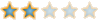Montreal Math ClubMathematics students in Montreal - Étudiants de mathématiques à MontréalHomeCalendarFAQSearchMemberlistUsergroupsRegisterLog in

 Montreal Math Club :: Mathematics :: Calculus and Analysis Share

# Exam questionAuthorMessage

PythagorasPosts : 17
Join date : 2009-11-09Subject: Exam questionFri Nov 13, 2009 4:50 pm Hi. This is Jim. This is the problem that I showed Bruno last night from an old Concordia Ph. D. Comprehensive Examination. Question 1.(b)Prove: If f(x) -> + infinity and f'(x) -> 0 as x -> infinity then f is not a quotient of two polynomials.The solution I have seems too simple so I'd like to see if there is a proof using the logarithmic derivative of f that Bruno suggested.Posts : 184
Join date : 2009-09-15
Age : 32
Location : the infinite, frictionless plane of uniform densitySubject: Re: Exam questionFri Nov 13, 2009 5:37 pm Hello Jim!The solution I suggested does not work after all. Indeed the log. derivative of a quotient of polynomial goes to 0 at infinity, so we can't use that!I would go like this : suppose f(x)=p(x)/q(x) is a quotient of polynomials. Then deg(p(x))>deg(q(x)), because of the first assumption. But f'(x)=(p'(x)q(x)-p(x)q'(x))/q(x)^2 is also a quotient of polynomials. The only way for this to converge to 0 at infinity is if we have : deg(p'(x)q(x)-p(x)q'(x))deg(q(x)).What's your solution?Posts : 184
Join date : 2009-09-15
Age : 32
Location : the infinite, frictionless plane of uniform densitySubject: Re: Exam questionFri Nov 13, 2009 7:48 pm Here is my solution to #2a. Gave me some trouble!Let C be the set of conjugate subgroups of H, and N be the normalizer of H in G. Then we can rephrase the problem by saying that if G is the union of the elements of C, then H=G.If |C|>1 then we must have |C| > [G : H], because every element of C has order |H|, but since |C|>1 the union is not disjoint - the elements of C have the identity in common.A well known theorem states that |C| = [G : N]. Therefore [G : N] > [G : H], i.e. |G|/|N| > |G|/|H|, or |H|>|N|. This is absurd, because H is contained in N. Therefore |C|=1, H is normal in G and H=G.PythagorasPosts : 17
Join date : 2009-11-09Subject: Re: Exam questionSun Nov 15, 2009 2:05 pm Yes, that is exactly my solution. I haven't tried the group theory question yet though. So I will try it and see if I get the same answer.EuclidPosts : 95
Join date : 2009-09-15
Age : 57
Location : AlexandriaSubject: Re: Exam questionFri Feb 05, 2010 5:26 pm #2a is exercise 24, page 131 in Abstract Algebra Dummit & Foote.I'd say : if a subgroup contains a representative for each conjugacy class, it must be the entire group.Subject: Re: Exam questionExam questionPage 1 of 1
 Similar topics» Masterchef/ Chefs in general- Question
» Women Inventors Exam- Mostaganem
» 3rd Term exam
» dreaming i need to retake my college entry exam
» First Term Exam - Crossword Puzzle

Permissions in this forum:You cannot reply to topics in this forumJump to: Select a forum||--Math Club|   |--Mathematical Sundays|   |--Suggestions|   |--Introduce yourself|   |--Mathematics|   |--Problem Solving|   |   |--Solved problems|   |   |   |--Calculus and Analysis|   |--Combinatorics|   |--Geometry|   |--Number Theory|   |--Probabilities and statistics|   |--Set theory and Topology|   |--Online Resources|   |--Chit chat    |--The Water Cooler    |--Mathematical poems